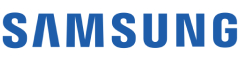New update is available. Click here to update.
Topics

# Second Largest Number

Easy0/40
Average time to solve is 23m## Problem statement

You have been given an array ‘a’ of ‘n’ unique non-negative integers.

Find the second largest and second smallest element from the array.

Return the two elements (second largest and second smallest) as another array of size 2.

Example :
``````Input: ‘n’ = 5, ‘a’ = [1, 2, 3, 4, 5]
Output: [4, 2]

The second largest element after 5 is 4, and the second smallest element after 1 is 2.
``````
Detailed explanation ( Input/output format, Notes, Images )
Sample Input 1 :
``````4
3 4 5 2
``````
Sample Output 1 :
``````4 3
``````
Explanation For Sample Input 1 :
``````The second largest element after 5 is 4 only, and the second smallest element after 2 is 3.
``````
Sample Input 2 :
``````5
4 5 3 6 7
``````
Sample Output 2 :
``````6 4
``````
Expected Time Complexity:
``````O(n), Where ‘n’ is the size of an input array ‘a’.
``````
Constraints:
``````2 ≤ 'n' ≤ 10^5
0 ≤ 'a'[i] ≤ 10^9

Time limit: 1 sec
``````Console